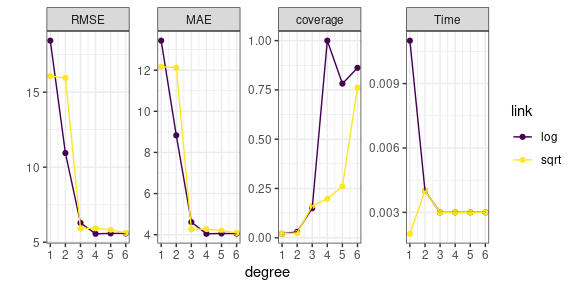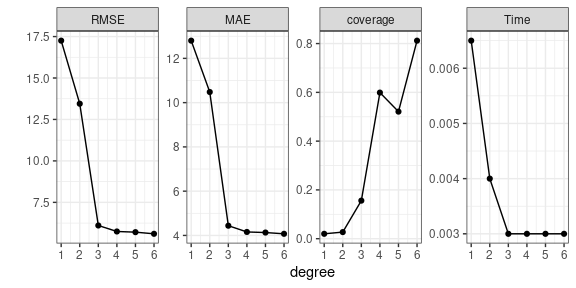# testarguments

#### 22-05-2021

Prediction algorithms typically have at least one user-specified argument that can have a considerable effect on predictive performance. Specifying these arguments can be a tedious task, particularly if there is an interaction between argument levels. This package is designed to neatly and automatically test and visualise the performance of a user-defined prediction algorithm over an arbitrary number of arguments. It includes functions for testing the predictive performance of an algorithm with respect to a set of user-defined diagnostics, visualising the results of these tests, and finding the optimal argument combinations for each diagnostic. The typical workflow involves:

1. Defining a prediction algorithm that uses training data and predicts over a testing set.
2. Defining a set of diagnostics as a function that quantify the performance of the predictions over the testing set.
3. Using test_arguments() to train and test the prediction algorithm over a range of argument values. This creates an object of class testargs, the central class definition of the package.
4. Visualising the predictive performance using plot_diagnostics().
5. Computing the optimal combination of arguments for each diagnostic using optimal_arguments().

## Example: Poisson regression with glm()

We demonstrate testarguments using a Poisson regression example, where the prediction algorithm is the generalised linear model (GLM) implemented with glm().

### Setup

First, load testarguments and simulate training and testing data. The data is Poisson distributed, with conditional expectation constructed by passing a fourth-order polynomial function of a covariate x through an exponential link function.

library("testarguments")
RNGversion("3.6.0"); set.seed(1)
n  <- 1000                                    # sample size
x  <- seq(-1, 1, length.out = n)              # covariates
Y  <- 3 + 2 * x * (x - 1) * (x + 1) * (x - 2) # linear predictor
mu <- exp(Y)                                  # mean of data
Z  <- rpois(n, mu)                            # simulate data
df       <- data.frame(x = x, Z = Z, mu = mu)
train_id <- sample(1:n, n/2, replace = FALSE)
df_train <- df[train_id, ]                    # training set
df_test  <- df[-train_id, ]                   # testing set

Next, we define a wrapper function that trains our prediction algorithm with df_train and predicts over df_test. In this example, the arguments that we wish to test are the link function, and the degree of the polynomial used to model the conditional expectation; these must be included as formal arguments in the wrapper function.

pred_fun <- function(df_train, df_test, degree, link) {

M <- glm(Z ~ poly(x, degree), data = df_train,

## Predict over df_test
pred <- as.data.frame(predict(M, df_test, type = "link", se.fit = TRUE))

## Compute response level predictions and 90% prediction interval
inv_link <- family(M)$linkinv fit_Y <- pred$fit
se_Y  <- pred\$se.fit
upr_Z = inv_link(fit_Y + 1.645 * se_Y),
lwr_Z = inv_link(fit_Y - 1.645 * se_Y))

return(pred)
}

Now, we define a diagnostic function, which should return a named vector. Here, we use the root-mean-squared error (RMSE), the mean-absolute error (MAE), and the coverage.

diagnostic_fun <- function(df) {
with(df, c(
RMSE     = sqrt(mean((Z - fit_Z)^2)),
MAE      = mean(abs(Z - fit_Z)),
coverage = mean(lwr_Z < mu & mu < upr_Z)
))
}

### Test the arguments of the prediction algorithm

Now we enter the main purpose of the package; to compute the user-defined diagnostics over a range of argument combinations. This is done using test_arguments(), which takes the prediction function, training data, testing data, the diagnostic fun, and a list of arguments to test. In this case, we will test the model using a polynomial of degree 1 to 6, and using the log and square-root link function.

testargs_object <- test_arguments(
pred_fun, df_train, df_test, diagnostic_fun,
arguments = list(degree = 1:6, link = c("log", "sqrt"))
)

### Visualise predictive performance

It is informative to visualise the predictive performance across all argument combinations; this is done using plot_diagnostics().

plot_diagnostics(testargs_object)Using various aesthetics, plot_diagnostics() can visualise the performance of all combinations of up to 4 different arguments simultaneously. In the above plot, we can see that the predictive performance is not particularly sensitive to the choice of link function. We can focus on a subset of arguments using the argument focused_args. By default, this averages out the arguments not included in focused_args.

plot_diagnostics(testargs_object, focused_args = "degree")### Optimal arguments

The function optimal_arguments() computes the optimal arguments from a testargs object. The measure of optimality is typically diagnostic dependent; for example, we typically wish to minimise the RMSE and run time, but we want coverage to be as close to the purported value as possible. Hence, optimal_arguments() allows one to set the optimality criterion individually for each diagonstic rule.

optimal_arguments(
testargs_object,
optimality_criterion = list(coverage = function(x) which.min(abs(x - 0.90)))
)
#>          degree link      RMSE       MAE coverage  Time
#> RMSE          4  log  5.559396  4.042814    1.000 0.003
#> MAE           4  log  5.559396  4.042814    1.000 0.003
#> coverage      6  log  5.586129  4.055873    0.862 0.003
#> Time          1 sqrt 16.070260 12.163413    0.020 0.002

In the output, the row names specify the diagnostic that is optimised by the corresponding combination of arguments. We can see that the RMSE and MAE are optimised when the degree of the polynomial function is 4 and the log link function is used; recall that these are the true values. In contrast, the coverage is optimised when the degree of the polynomial function is 6.